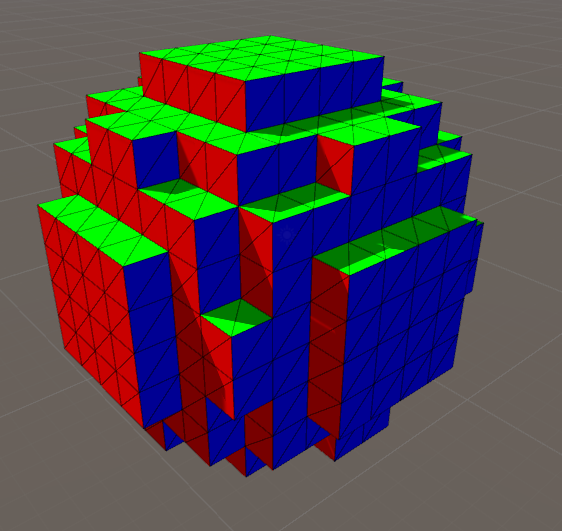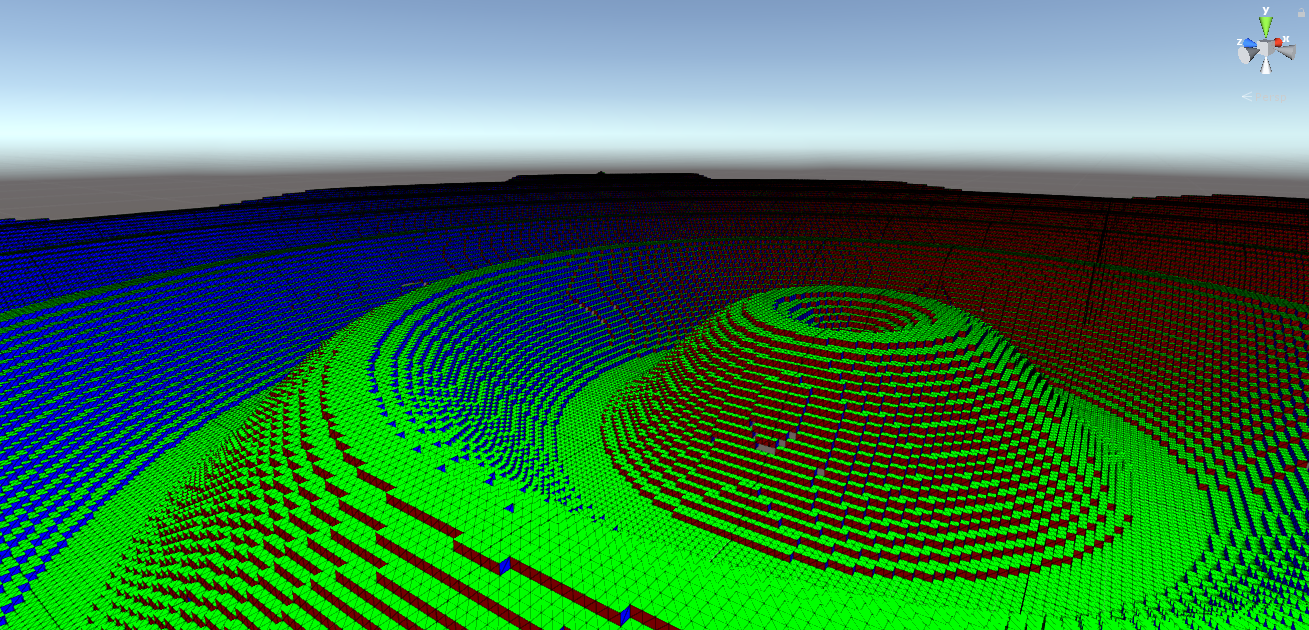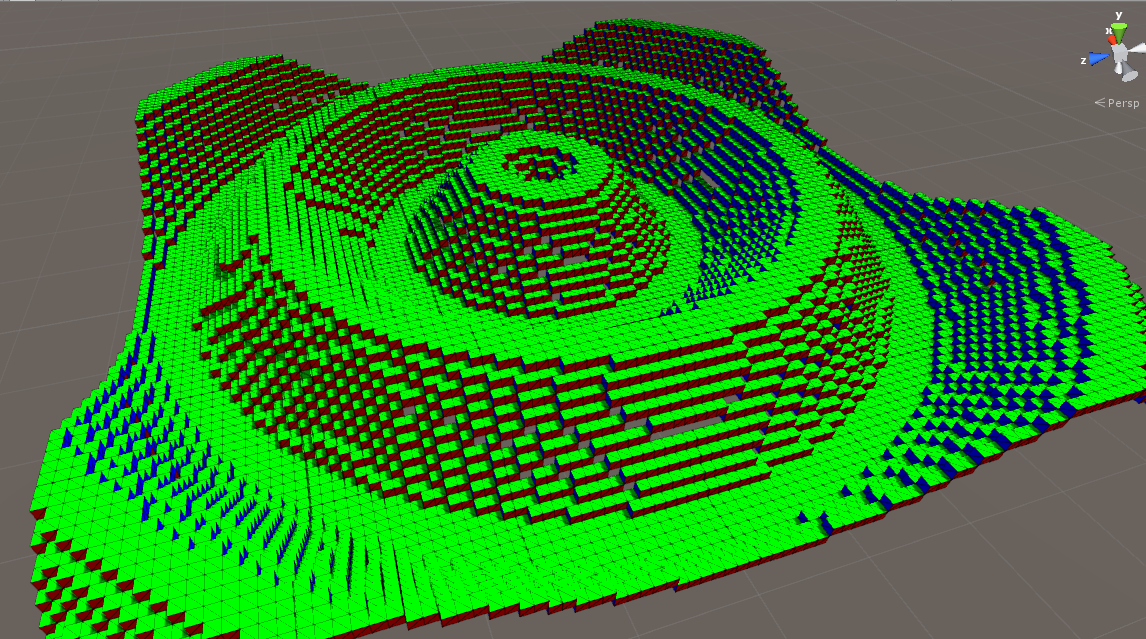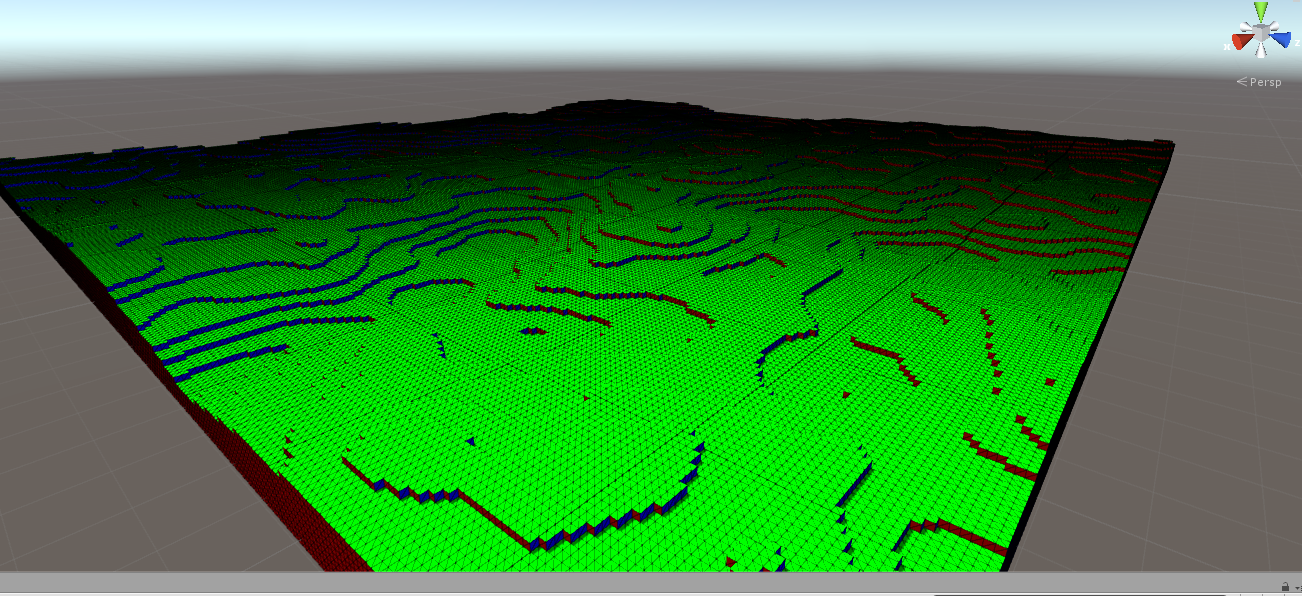# Procedural World - Introduction

In a previous post I mentioned I’m starting a new procedural terrain project. Welp. Here it is.

This project is intended as a playground for procedurally generated worlds. I’d like to generate terrain (hills, mountains, rivers, roads, biomes), villages, citys and things like that. Pretty much anything you would find in the world.

I’m kind of going for a fantasy/medival RPG type thing. But no specific gameplay mechanics in mind, I’m more interested in generating the world at the moment. I figure this could be used as the basis for different types of games in the future.

## Iterative Design

Something a get stuck on a lot is spending way too much time thinking of the best way to go about solving a problem and not enough time coding up front.

For this project especially, where I don’t really have specific constraints or scope, there isn’t a lot of advantage to planning everything from the get go.

So I’m focusing on adding one step at a time and worrying about optimiation later.

## First Stages

The first step was to simple draw a cube. Now I’m not exactly going for a MineCraft clone here but cubes/blocks are a easy to reason about and easy to setup. To the first thing I did was generate a simple cube mesh.

That looks something like this:

using System.Collections;
using System.Collections.Generic;
using UnityEngine;

[RequireComponent(typeof(MeshFilter), typeof(MeshRenderer))]
public class CubeMesh : MonoBehaviour
{
private Mesh mesh;

private const float CUBE_SIZE = 1f;
private const float HALF_CUBE_SIZE = CUBE_SIZE / 2f;

private static readonly Vector3[] cubeVertices =
{
// t b l r v f
new Vector3(-HALF_CUBE_SIZE, -HALF_CUBE_SIZE, -HALF_CUBE_SIZE), // lbn 0
new Vector3(-HALF_CUBE_SIZE, -HALF_CUBE_SIZE, HALF_CUBE_SIZE),  // lbf 1
new Vector3(HALF_CUBE_SIZE, -HALF_CUBE_SIZE, HALF_CUBE_SIZE),   // rbf 2
new Vector3(HALF_CUBE_SIZE, -HALF_CUBE_SIZE, -HALF_CUBE_SIZE),  // rbn 3
new Vector3(-HALF_CUBE_SIZE, HALF_CUBE_SIZE, -HALF_CUBE_SIZE),  // ltn 4
new Vector3(-HALF_CUBE_SIZE, HALF_CUBE_SIZE, HALF_CUBE_SIZE),   // ltf 5
new Vector3(HALF_CUBE_SIZE, HALF_CUBE_SIZE, HALF_CUBE_SIZE),    // rtf 6
new Vector3(HALF_CUBE_SIZE, HALF_CUBE_SIZE, -HALF_CUBE_SIZE)    // rtn 7
};

private const int lbn = 0;
private const int lbf = 1;
private const int rbf = 2;
private const int rbn = 3;
private const int ltn = 4;
private const int ltf = 5;
private const int rtf = 6;
private const int rtn = 7;

private static readonly int[] faces =
{
// near
lbn, ltn, rtn,
lbn, rtn, rbn,

// far
rbf, rtf, ltf,
rbf, ltf, lbf,

// left
lbf, ltn, lbn,
lbf, ltf, ltn,

// right
rbn, rtn, rtf,
rbn, rtf, rbf,

// top
ltn, ltf, rtf,
ltn, rtf, rtn,

// bottom
lbf, lbn, rbn,
lbf, rbn, rbf
};

private void Awake()
{
mesh = new Mesh();
GetComponent<MeshFilter>().mesh = mesh;
}

private void Start()
{
List<Vector3> vertices = new List<Vector3>();

foreach (var v in  cubeVertices)
{
}

List<int> indices = new List<int>();

foreach(int i in faces)
{
}

mesh.vertices = vertices.ToArray();
mesh.triangles = indices.ToArray();

mesh.RecalculateNormals();
}
}


Also I applied this shader to color the mesh based on its normals.

Shader "Custom/NormalMap" {
Properties{
_MainTex("Texture", 2D) = "white" {}
}
Tags{ "RenderType" = "Opaque" }
CGPROGRAM

#pragma surface surf Lambert vertex:vert
struct Input {
float2 uv_MainTex;
float3 customColor;
};
void vert(inout appdata_full v, out Input o) {
UNITY_INITIALIZE_OUTPUT(Input,o);
o.customColor = abs(v.normal);
}

sampler2D _MainTex;
void surf(Input IN, inout SurfaceOutput o) {
o.Albedo = tex2D(_MainTex, IN.uv_MainTex).rgb;
o.Albedo *= IN.customColor;
}
ENDCG
}

Fallback "Diffuse"
}


The next obivious step was to create Chunks. Chunks are groupings or blocks and are responsible for creating the block mesh.

Now due to some previous research I know that I want to create the meshes using a 3D scalar field (just an array of floats). So I started with generating some simple scalar fields to test the mesh creation. For example:Now this is a single chunks. I know I’ll need multiple chunks eventually so that is the next step.

I created a FieldGenerator interface and multiple implementers to generate various patterns I could switch between.

This one generates a 2D sine wave.


using System;
using System.Collections.Generic;
using UnityEngine;

class SineFieldGenerator : FieldGenerator
{
void FieldGenerator.Generate(Field field, Transform transform)
{
// chunk world position
Vector3 p = transform.position;

float a = (float)field.Y / 3f;
const float f = 0.25f;

field.ForEachXZ((x, z) => {
Vector3 bp = p + new Vector3(x * CubeMetrics.CUBE_SIZE, 0, z * CubeMetrics.CUBE_SIZE);

float m = Mathf.Sqrt(bp.x * bp.x + bp.z * bp.z);
float y = a * Mathf.Sin(m * f) + a;
y = Mathf.Clamp(y, 0, field.Y);

field.Set(x, (int)y, z, 1);
});

}
}## Height Maps

To start generating terrain it makes sense to start simple. I implemented a Perlin Noise height map field generator.I’m currently working on adding infinite terrain.